A Python library of standardized optimization test functions

## Project description## Installation

There are a couple ways in which you can use this library. The first and probably the easiest is by using pip and PyPi:

pip install landscapes


You can also install directly from this git repo:

pip install git+https://github.com/nathanrooy/landscapes


wget https://github.com/nathanrooy/landscapes/archive/master.zip
unzip master.zip
cd landscapes-master


## Available functions from: single_objective

function name method dimensions
Ackley ackley() 2
Ackley N.2 ackley_n2() 2
Adjiman adjiman() 2
AMGM amgm() n
Bartels Conn bartels_conn() 2
Bird bird() 2
Beale beale() 2
Bent Cigar bent_cigar() n
Bohachevsky N.1 bohachevsky_n1() 2
Bohachevsky N.2 bohachevsky_n2() 2
Bohachevsky N.3 bohachevsky_n3() 2
Booth booth() 2
Branin branin() 2
Brent brent() 2
Brown brown() n
Bukin n6 bukin_n6() 2
3-Hump Camel camel_hump_3() 2
6-Hump Camel camel_hump_6() 2
Carrom Table carrom_table() 2
Chichinadze chichinadze() 2
Chung Reynolds chung_reynolds() n
Colville colville() 4
Cosine Mixture cosine_mixture() n
Cross-in-Tray cross_in_tray() 2
Csendes csendes() n
Cube cube() 2
Damavandi damavandi() 2
Deckkers-Aarts deckkers_aarts() 2
Dixon & Price dixon_price() n
Drop Wave drop_wave() 2
Easom easom() 2
Eggholder eggholder() 2
Exponential exponential() n
Freudenstein Roth freudenstein_roth() 2
Goldstein–Price goldstein_price() 2
Griewank griewank() n
Himmelblau himmelblau() 2
Hölder table holder_table() 2
Hosaki hosaki() 2
Keane keane() 2
Leon leon() 2
Lévi function N.13 levi_n13() 2
Matyas matyas() 2
Michalewicz michalewicz n
McCormick mccormick() 2
Parsopoulos parsopoulos() 2
Pen Holder pen_holder() 2
Plateau plateau() n
Qing qing() n
Quartic quartic() n
Rastrigin rastrigin() n
Rotated Hyper-Ellipsoid rotated_hyper_ellipsoid() n
Rosenbrock rosenbrock() n
Salomon salomon() n
Schaffer N.2 schaffer_n2() 2
Schaffer N.4 schaffer_n4() 2
Schwefel schwefel() n
Sphere sphere() n
Step step() n
Styblinski–Tang styblinski_tang() n
Sum of Different Powers sum_of_different_powers() n
Sum of Squares sum_of_squares() n
Trid trid() n
Tripod tripod() 2
Wolfe wolfe() 3
Zakharov zakharov() n

## Usage

As a simple example, let's use the Nelder-Mead method via SciPy to minimize the sphere function. We'll start off by importing the sphere function from Landscapes and the minimize method from SciPy.

>>> from landscapes.single_objective import sphere
>>> from scipy.optimize import minimize


Next, we'll call the minimize method using a starting location of [5,5].

>>> minimize(sphere, x0=[5,5], method='Nelder-Mead')


The output of which should look close to this:

 final_simplex: (array([[-3.33051318e-05, -1.93825710e-05],
[ 4.24925225e-05,  1.37129516e-05],
[ 3.09383247e-05, -4.04797876e-05]]), array([1.48491586e-09, 1.99365951e-09, 2.59579314e-09]))
fun: 1.4849158640215086e-09
message: 'Optimization terminated successfully.'
nfev: 80
nit: 44
status: 0
success: True
x: array([-3.33051318e-05, -1.93825710e-05])


## Function Reference - Single Objective

### Ackley function

from landscapes.single_objective import ackley

global minimum bounds usage
f(x=0,y=0)=0 -5.12 <= x, y <= 5.12 ackley([x,y])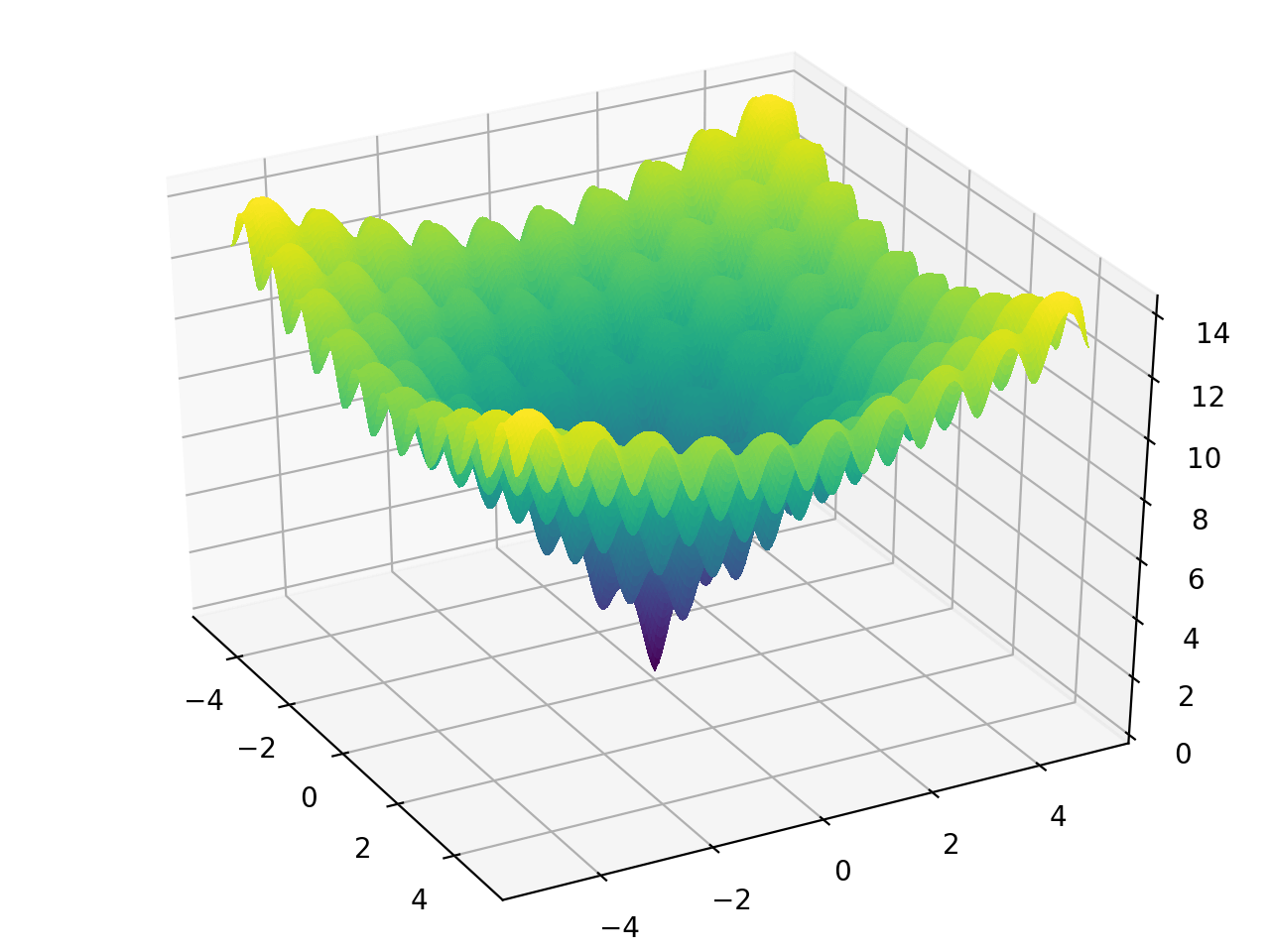### Beale function

from landscapes.single_objective import beale

global minimum bounds usage
f(x=3, y=0.5) = 0 -4.5 <= x, y <= 4.5 beale([x,y])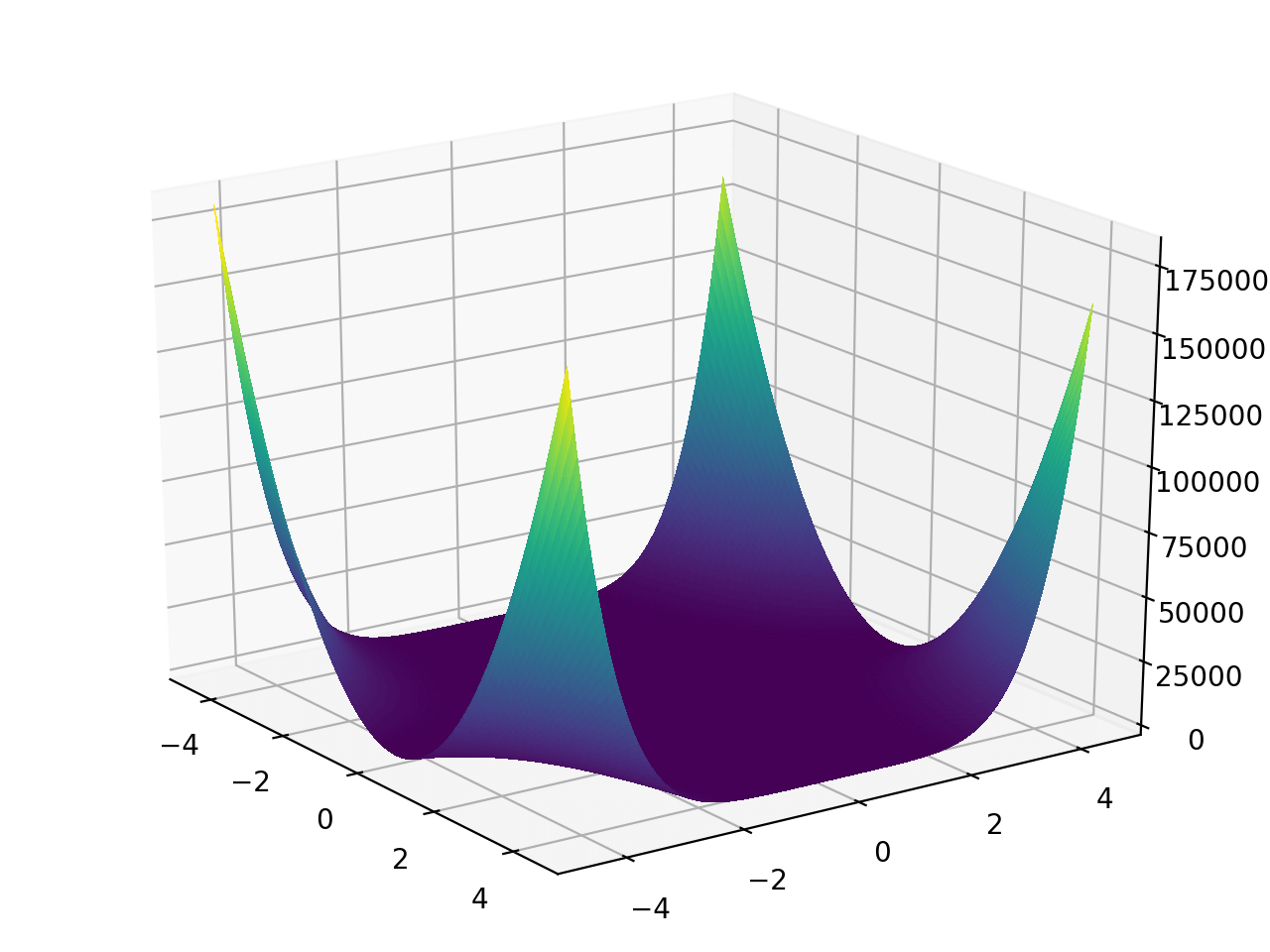### Booth function

from landscapes.single_objective import booth

global minimum bounds usage
f(x=1, y=3) = 0 -10 <= x, y <= 10 booth([x,y])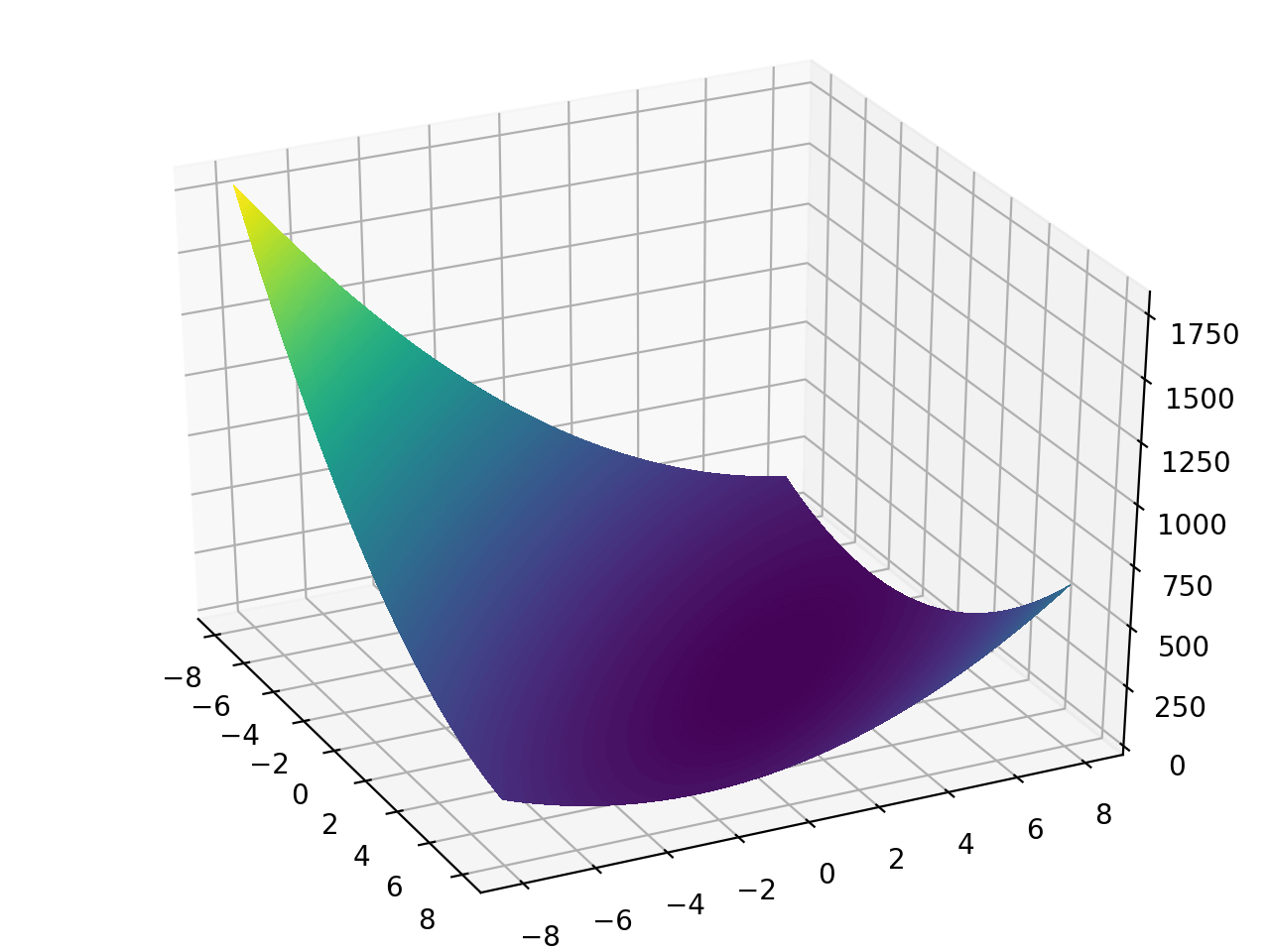### Bukin N.6 function

from landscapes.single_objective import bukin_n6

global minimum bounds usage
f(x=-10, y=1) = 0 -15 <= x <= -5
-3 <= y <= 3
bukin_n6([x,y])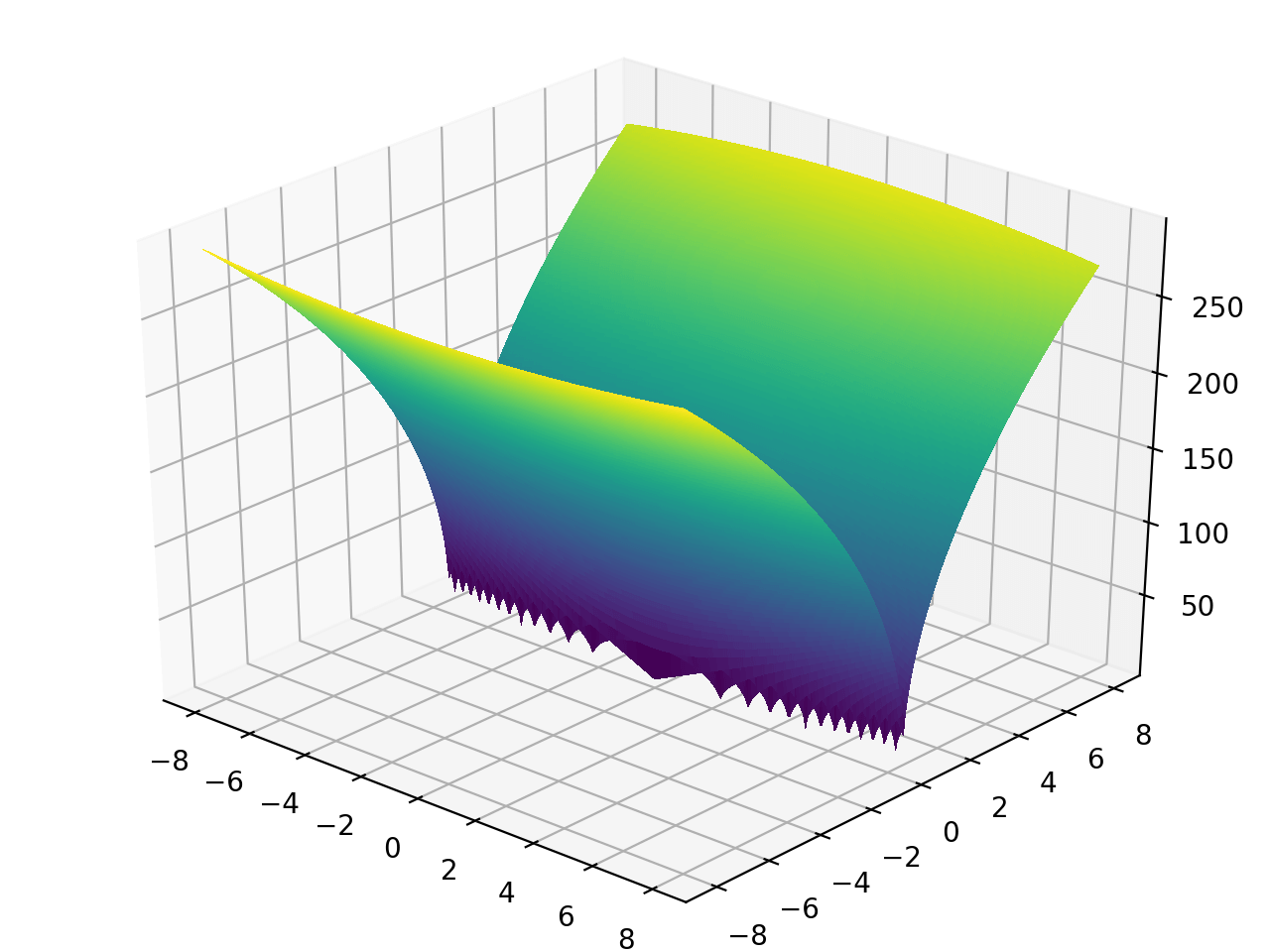### Cross-in-tray function

from landscapes.single_objective import cross_in_tray

global minimum(s) bounds usage
f(x=1.34941, y=-1.34941) = -2.06261
f(x=1.34941, y=1.34941) = -2.06261
f(x=-1.34941, y=1.34941) = -2.06261
f(x=-1.34941, y=-1.34941) = -2.06261
-10 <= x, y <= 10 cross_in_tray([x,y])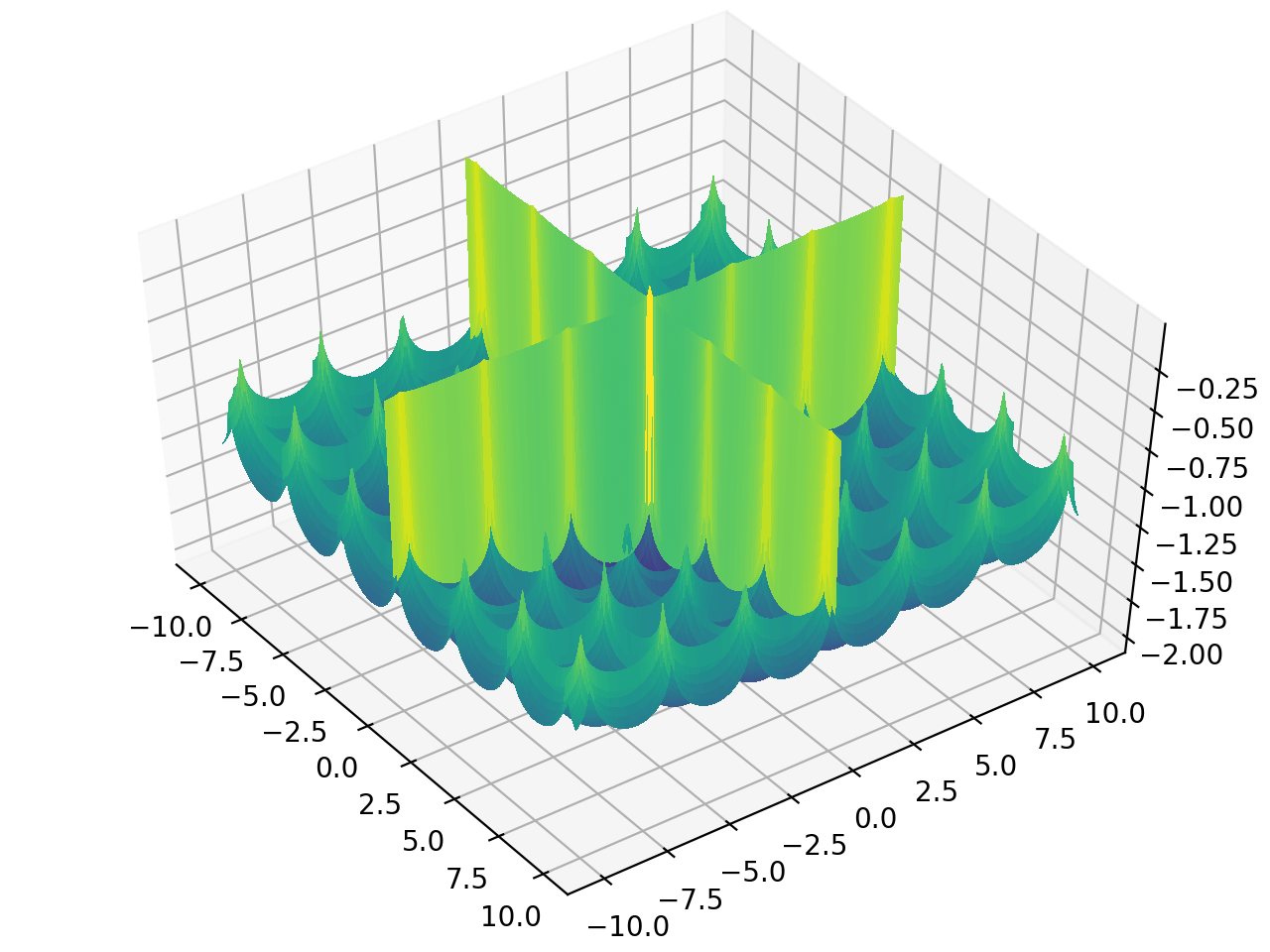### Easom function

from landscapes.single_objective import easom

global minimum bounds usage
f(x=pi, y=pi) = -1 -100 <= x, y <= 100 easom([x,y])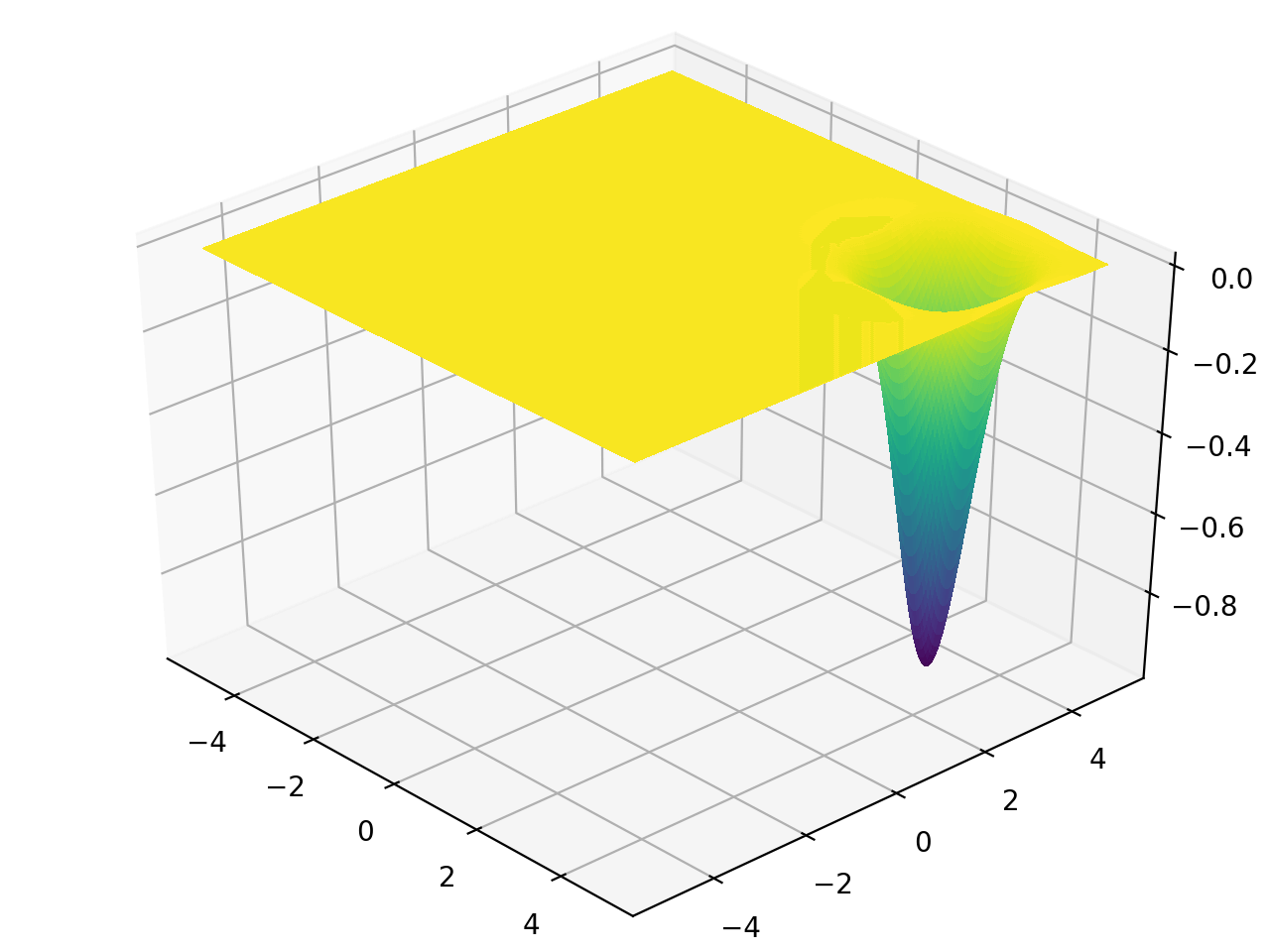### Eggholder function

from landscapes.single_objective import eggholder

global minimum bounds usage
f(x=512, y=404.2319) = -959.6407 -512 <= x, y <= 512 eggholder([x,y])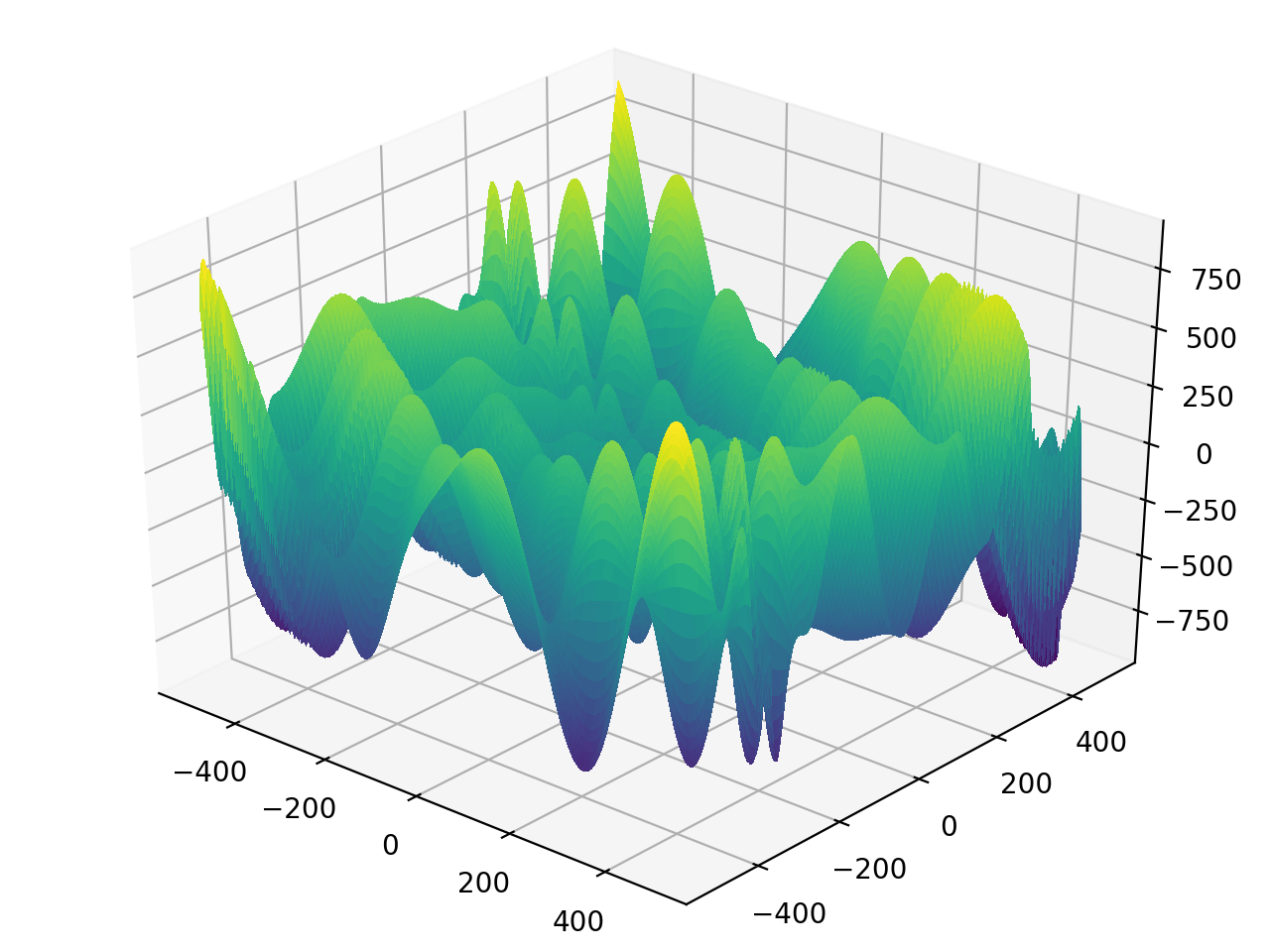### Goldstein–Price function

from landscapes.single_objective import goldstein_price

global minimum bounds usage
f(x=0, y=-1) = 3 -2 <= x, y <= 2 goldstein_price([x,y])### Himmelblau's function

from landscapes.single_objective import himmelblau

global minimum(s) bounds usage
f(x=3.0, y=2.0) = 0.0
f(x=-2.805118, y=3.131312) = 0.0
f(x=-3.779310, y=-3.283186) = 0.0
f(x=3.584428, y=-1.848126) = 0.0
-5 <= x, y <= 5 himmelblau([x,y])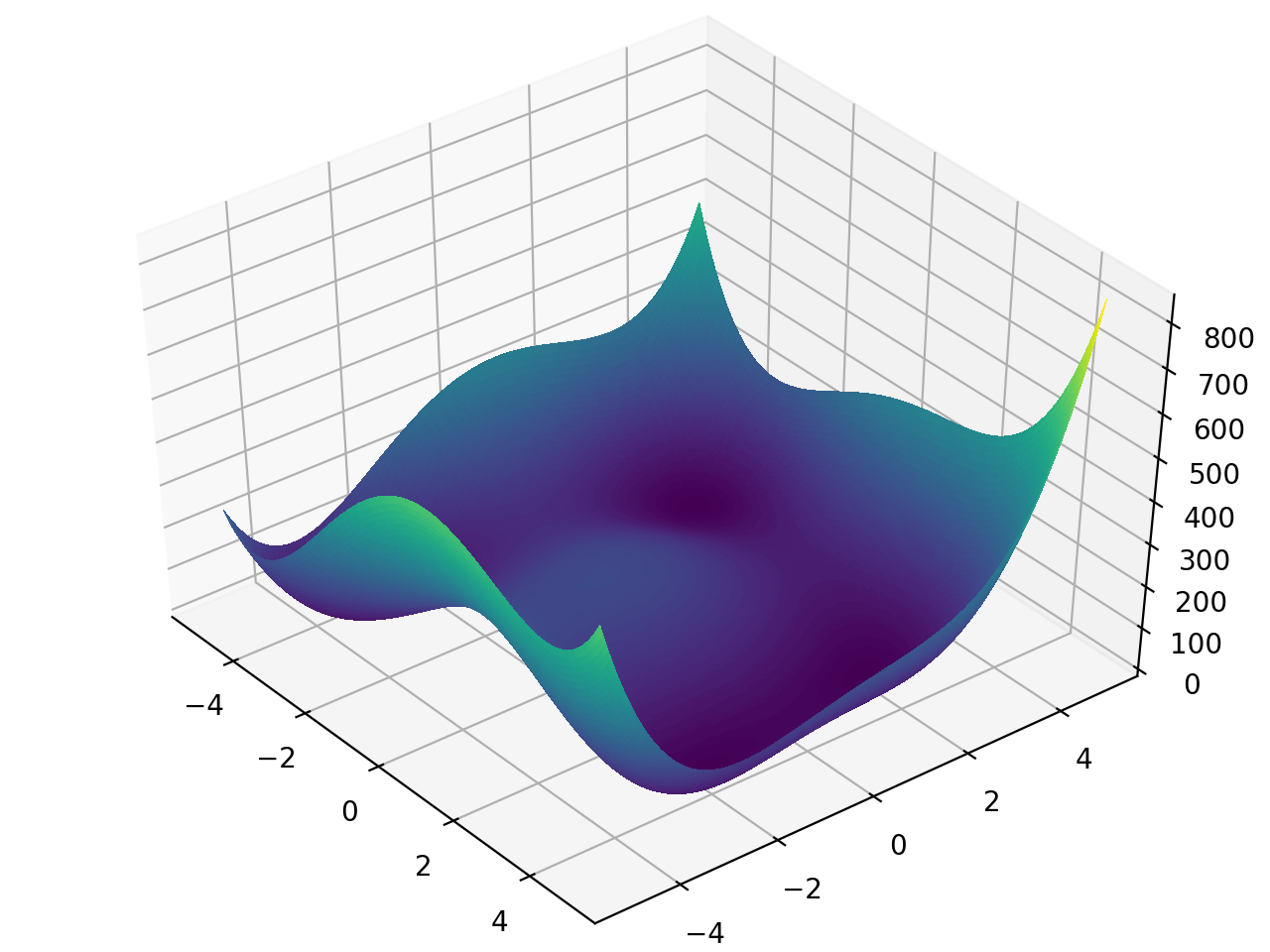### Hölder table function

from landscapes.single_objective import holder_table

global minimum(s) bounds usage
f(x=8.05502, y=9.66459) = -19.2085
f(x=-8.05502, y=9.66459) = -19.2085
f(x=8.05502, y=-9.66459) = -19.2085
f(x=-8.05502, y=-9.66459) = -19.2085
-10 <= x, y <= 10 holder_table([x,y])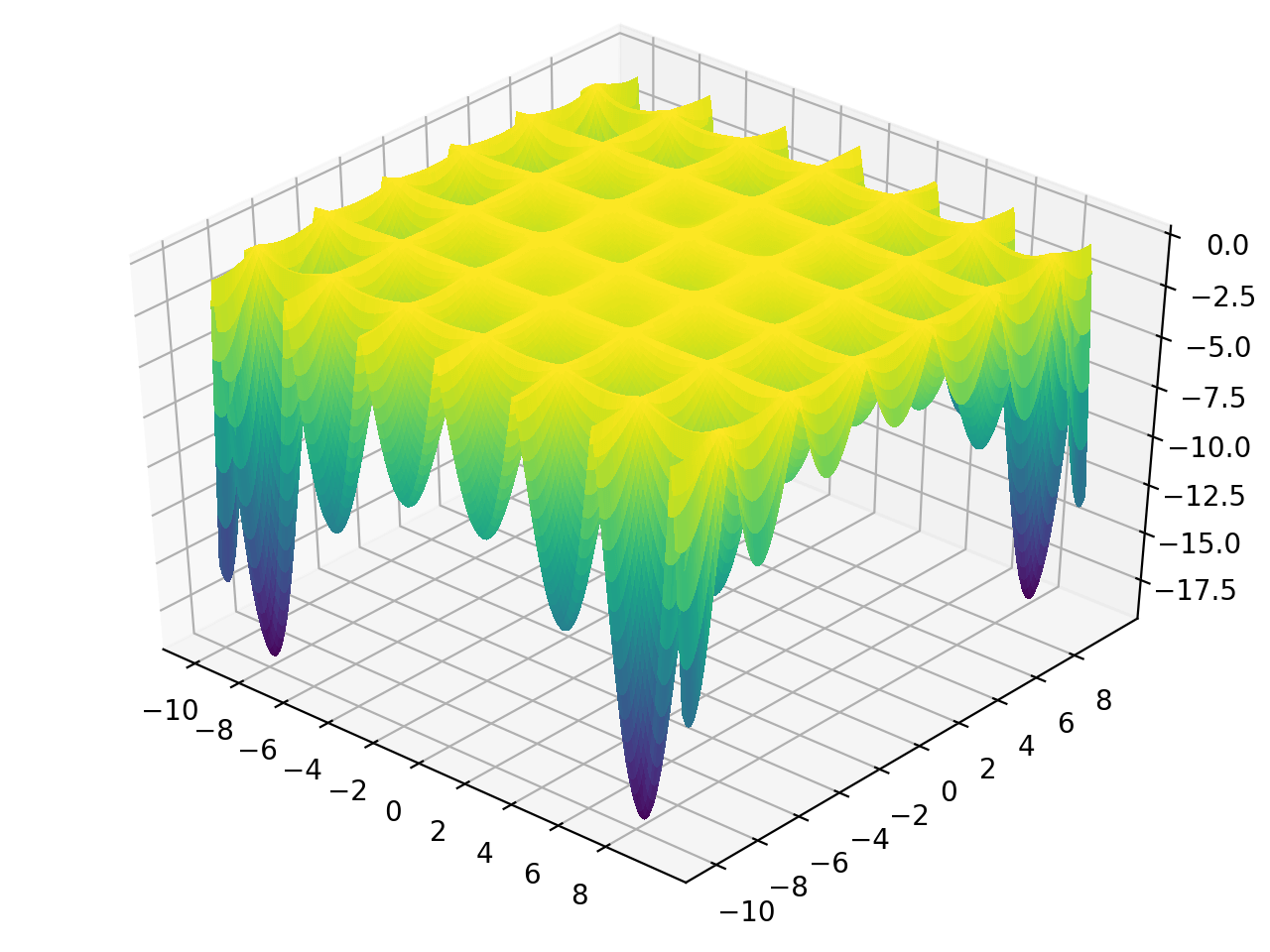### Lévi function N.13

from landscapes.single_objective import levi_n13

global minimum bounds usage
f(x=1, y=1) = 0 -10 <= x, y <= 10 levi_n13([x,y])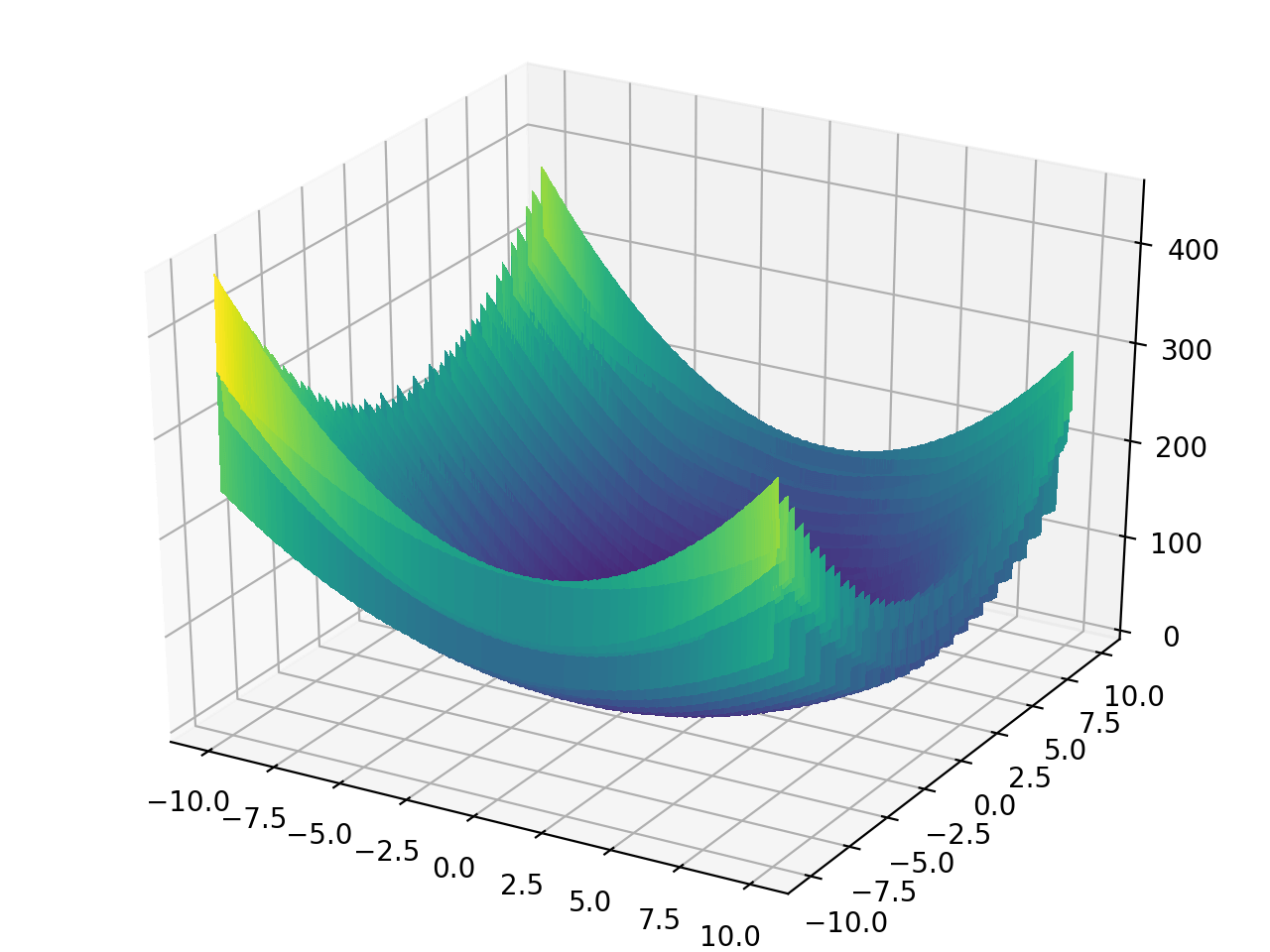### Matyas function

from landscapes.single_objective import matyas

global minimum bounds usage
f(x=0, y=0) = 0 -10 <= x, y <= 10 matyas([x,y])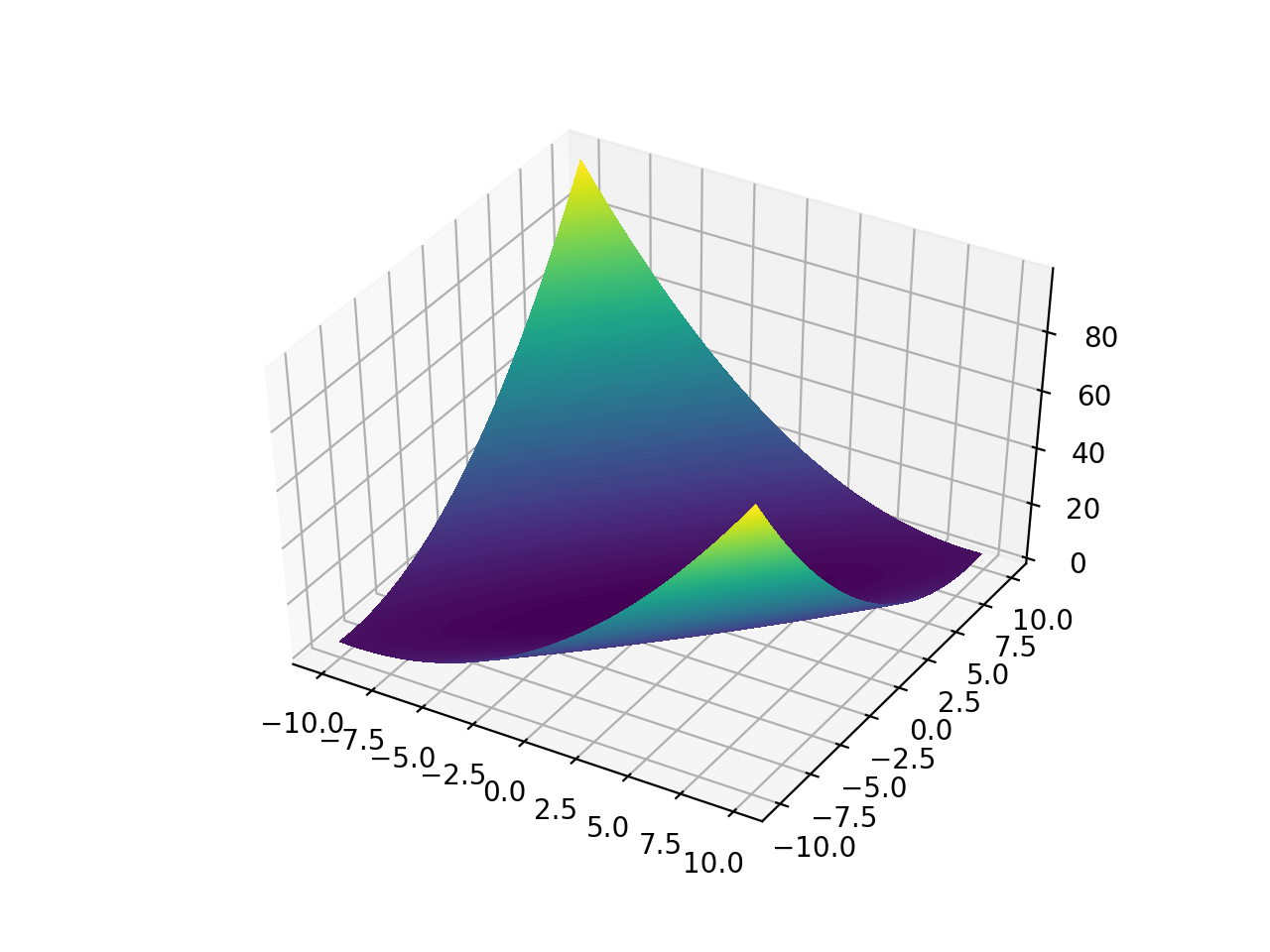### McCormick function

from landscapes.single_objective import mccormick

global minimum bounds usage
f(x=-0.54719, y=-1.54719) = -1.9133 -1.5 <= x <= 4
-3 <= y <= 4
mccormick([x,y])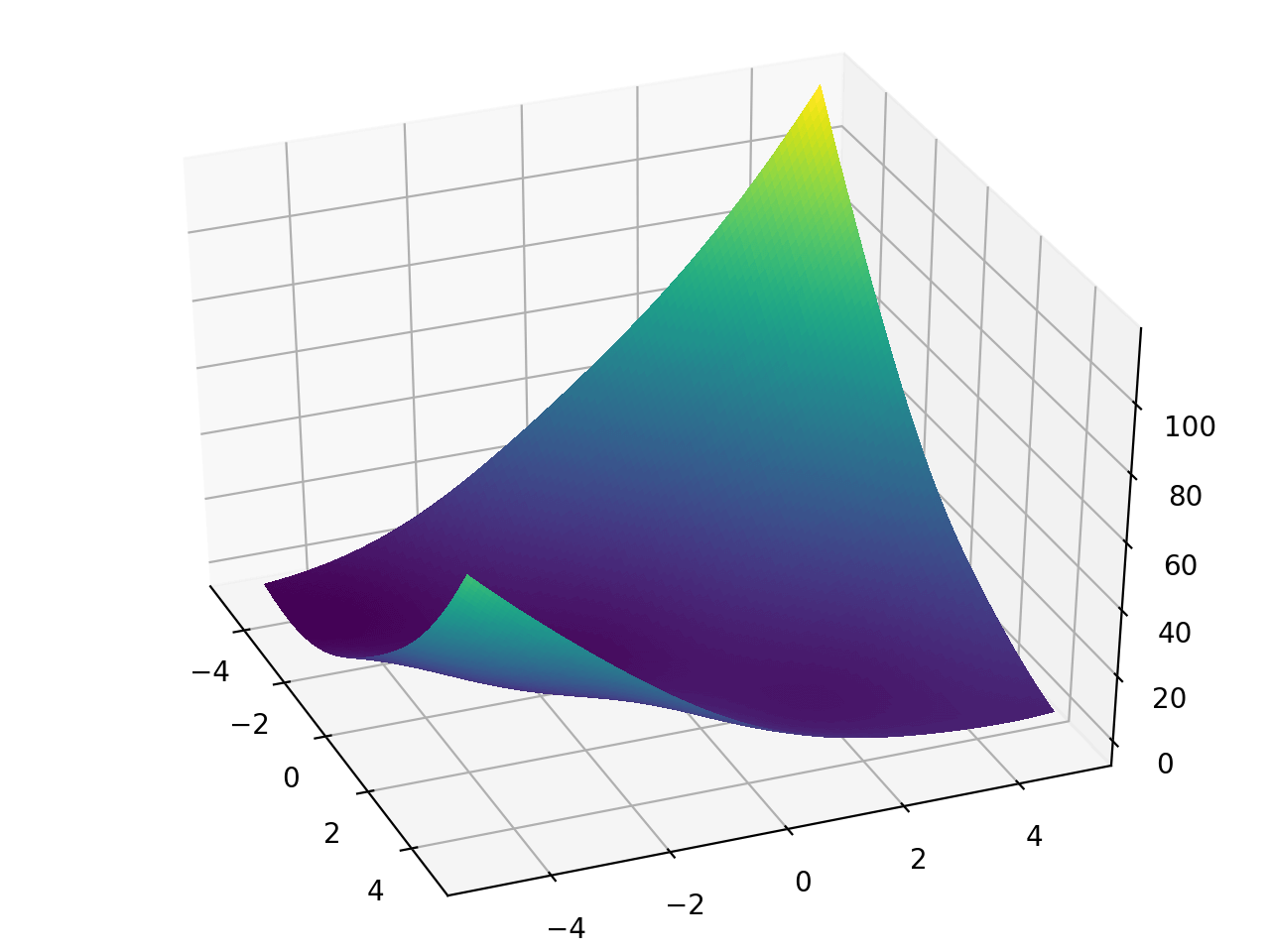### Rastrigin function

from landscapes.single_objective import rastrigin

global minimum bounds usage
f([0,...,0]) = 0 -5.12 <= x_i <= 5.12 rastrigin([x_1,...,x_n])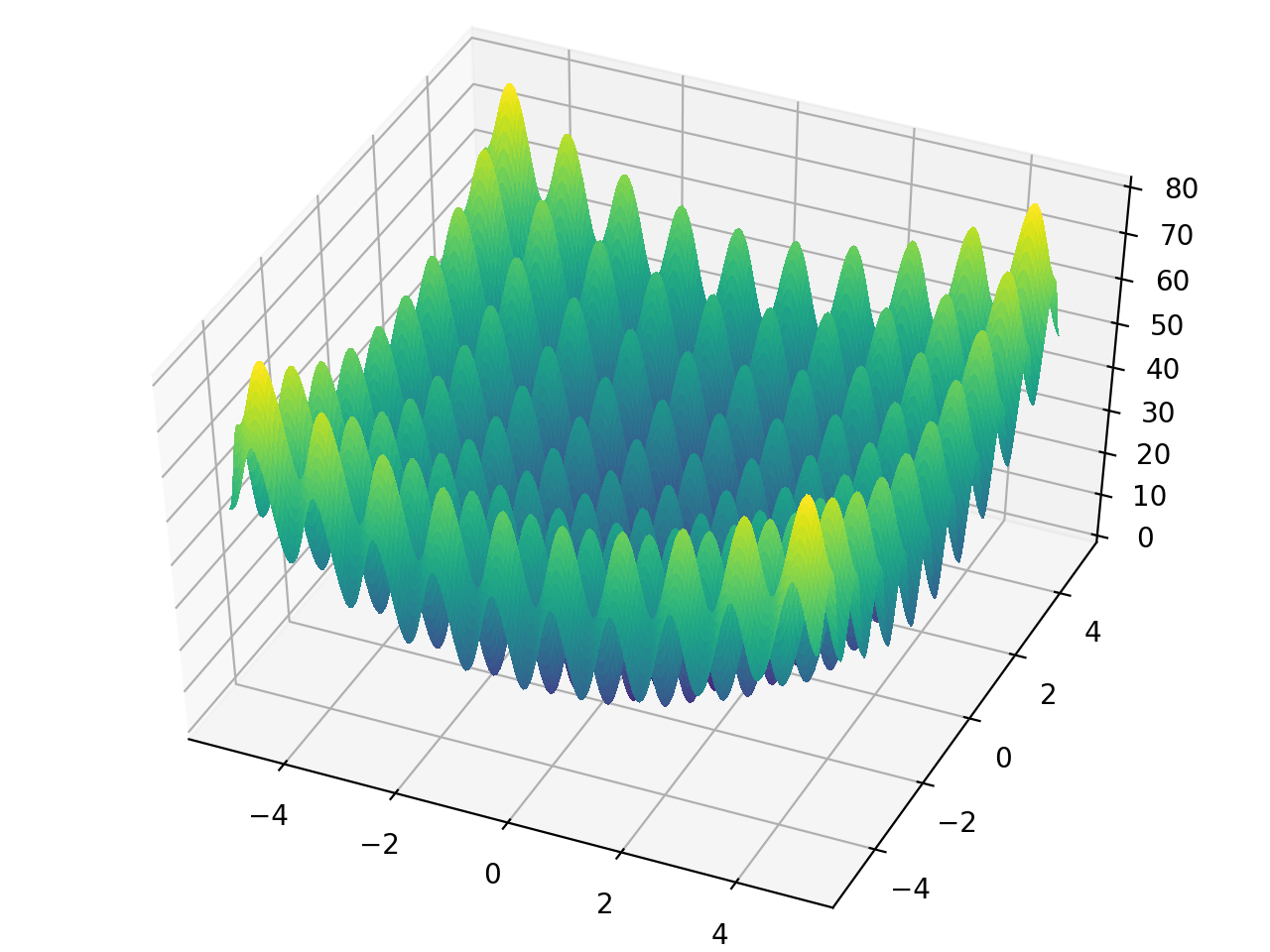### Rosenbrock function

from landscapes.single_objective import rosenbrock

global minimum bounds usage
f([1,...,1]) = 0 -inf <= x_i <= inf rosenbrock([x_1,...,x_n])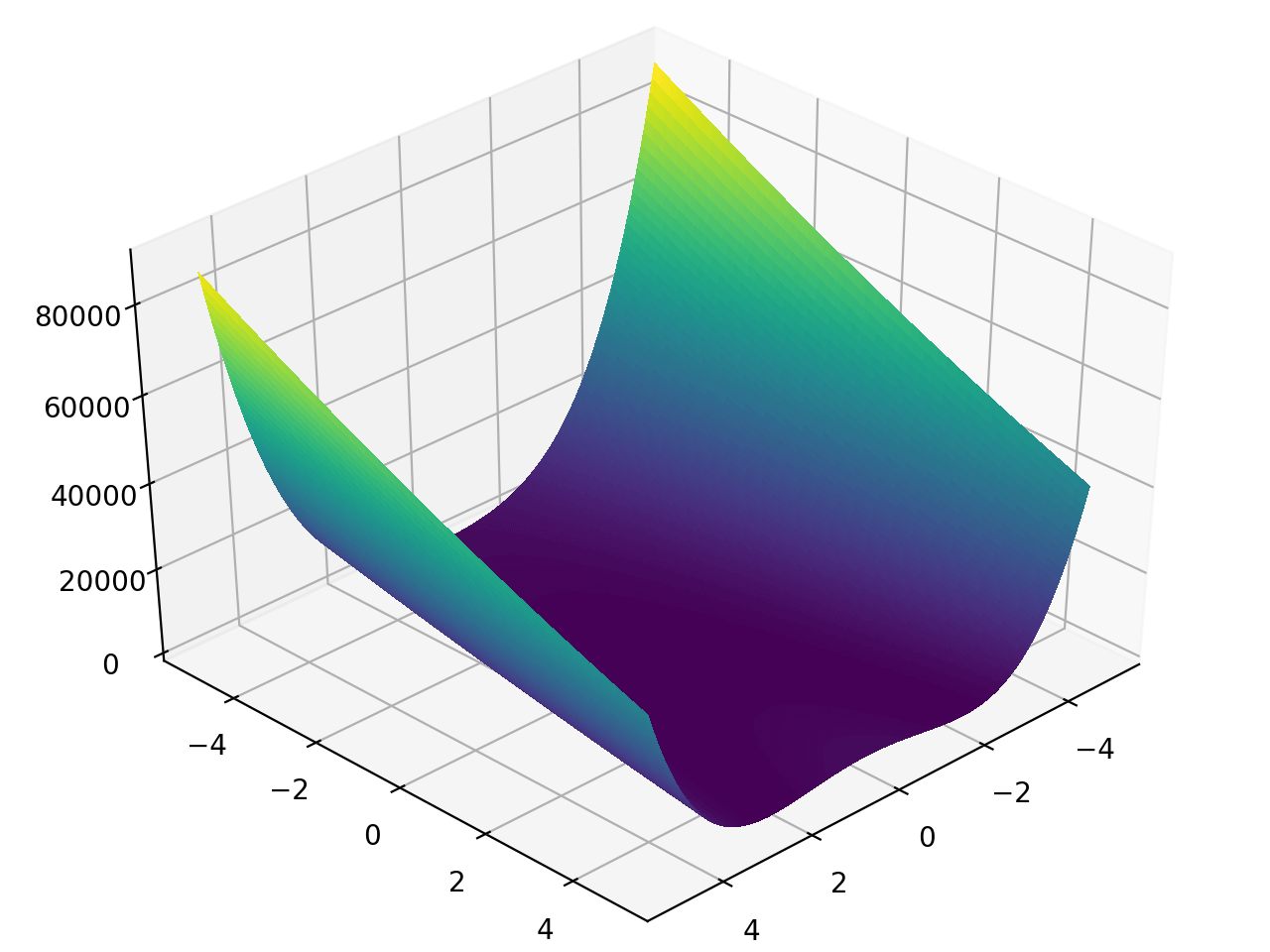### Schaffer function N.2

from landscapes.single_objective import schaffer_n2

global minimum bounds usage
f(x=0, y=0) = 0 -100 <= x, y <= 100 schaffer_n2([x,y])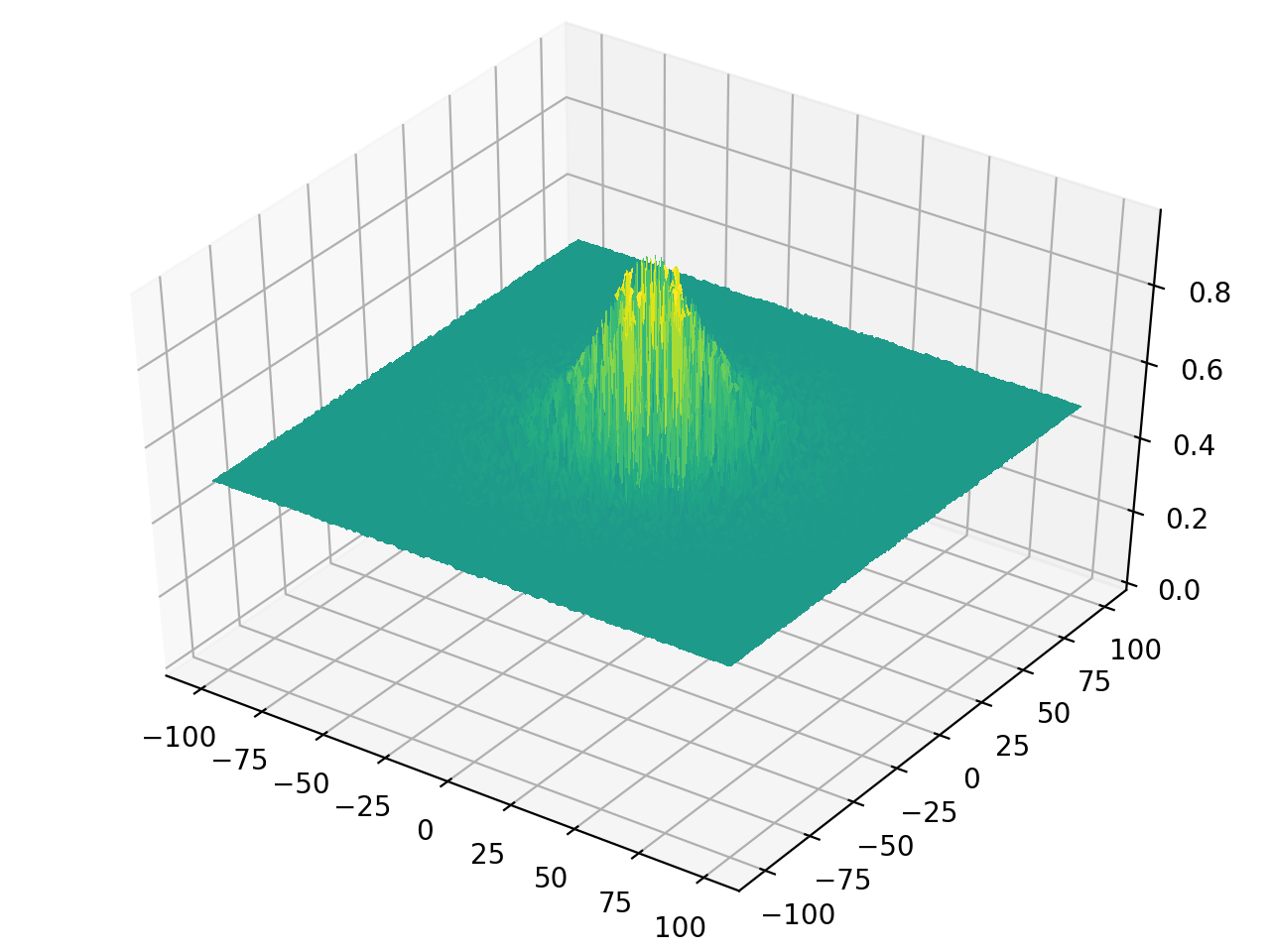### Schaffer function N.4

from landscapes.single_objective import schaffer_n4

global minimum bounds usage
f(x=0, y=1.25313) = 0.292579
f(x=0, y=-1.25313) = 0.292579
-100 <= x, y <= 100 schaffer_n4([x,y])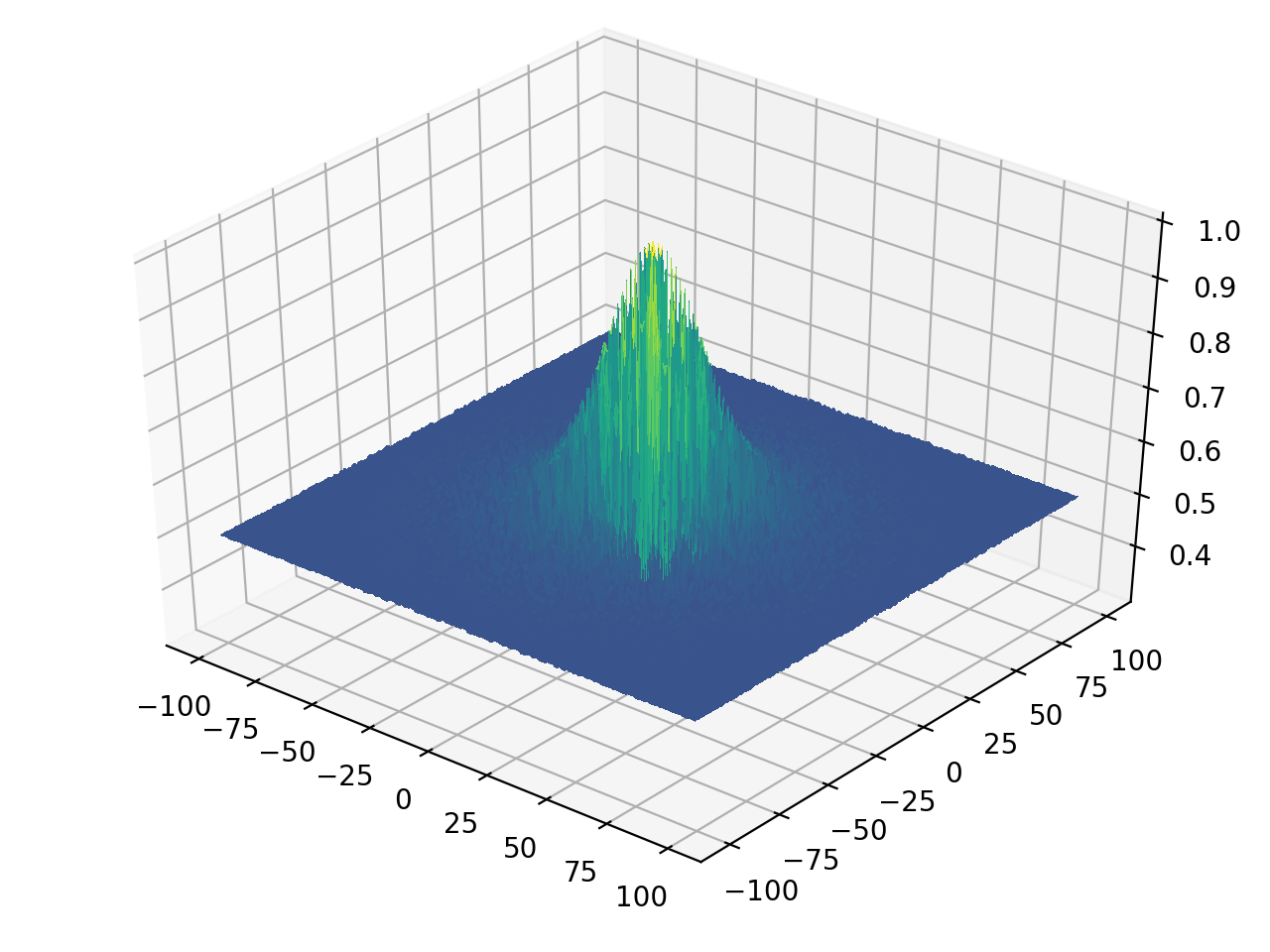### Sphere function

from landscapes.single_objective import sphere

global minimum bounds usage
f([0,...,0]) = 0 -inf <= x_i <= inf sphere([x_1,...x_n])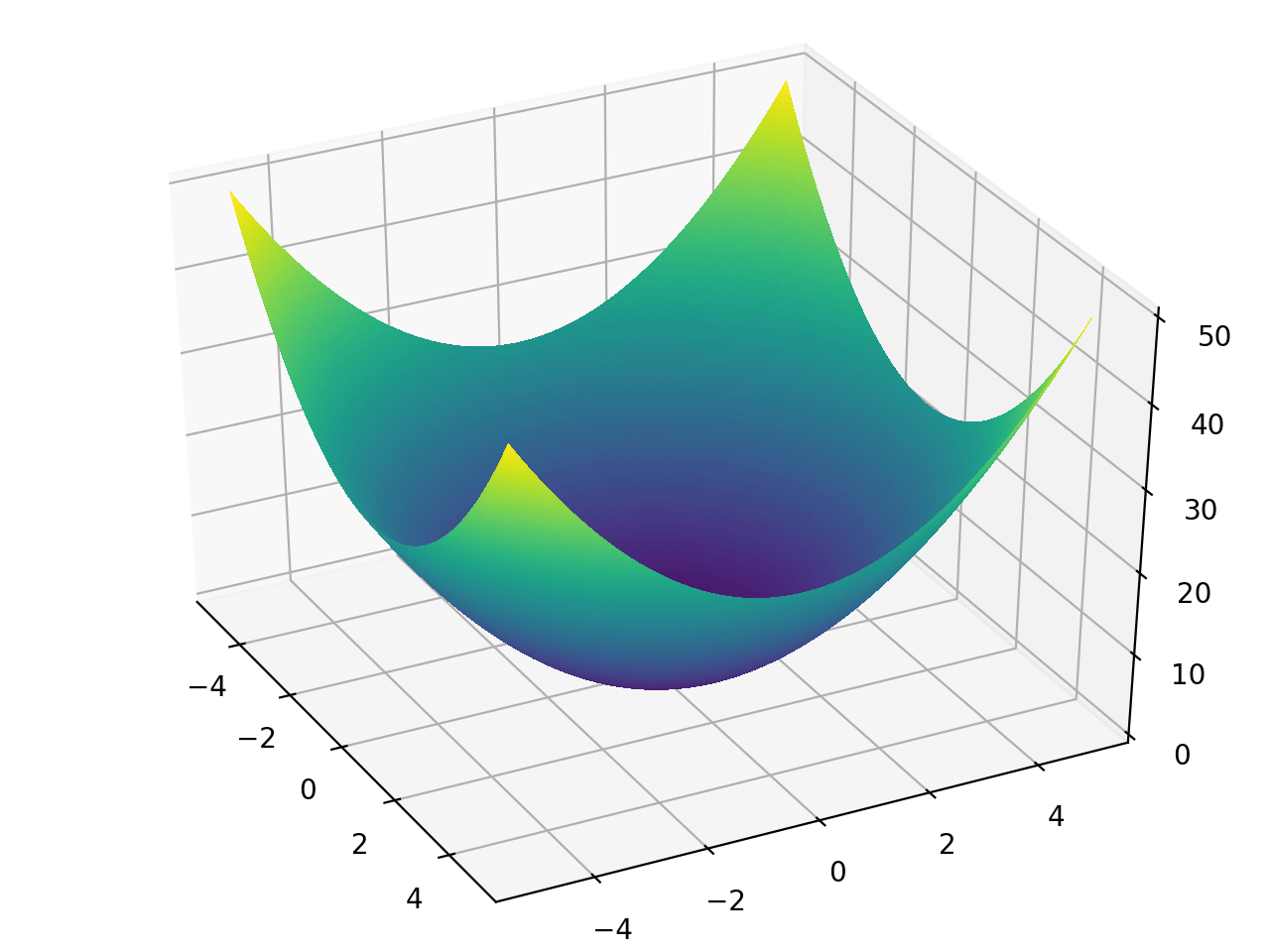### Styblinski–Tang function

from landscapes.single_objective import styblinski_tang

global minimum bounds usage
-39.16617n < f([-2.903534,...,-2.903534]) < -39.16616n -5 <= x_i <= 5 styblinski_tang([x_1,...x_n])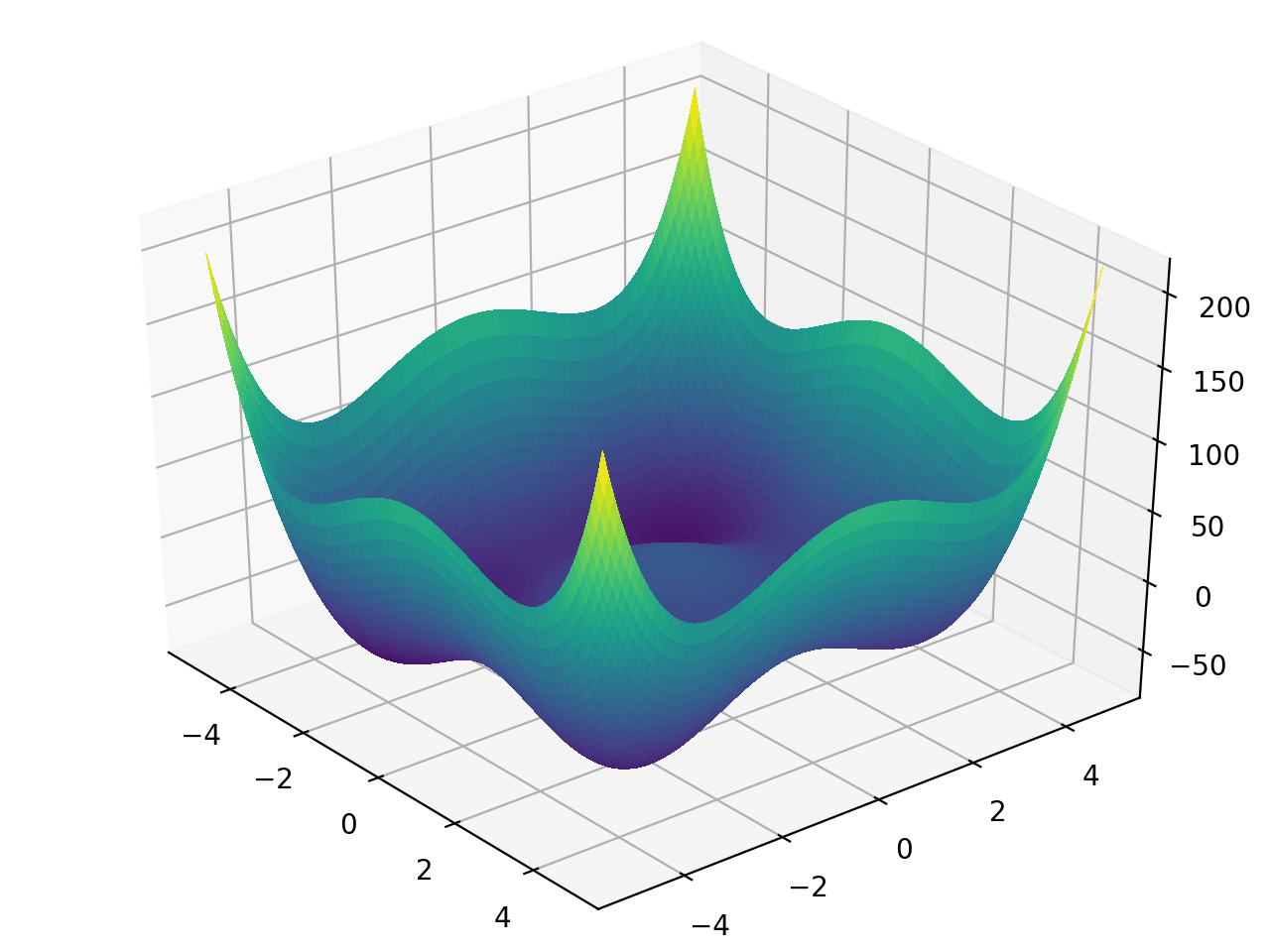### Three-hump camel function

from landscapes.single_objective import camel_hump_3

global minimum bounds usage
-f(x=0, y=0) = 0 -5 <= x_i <= 5 three_hump_camel([x,y])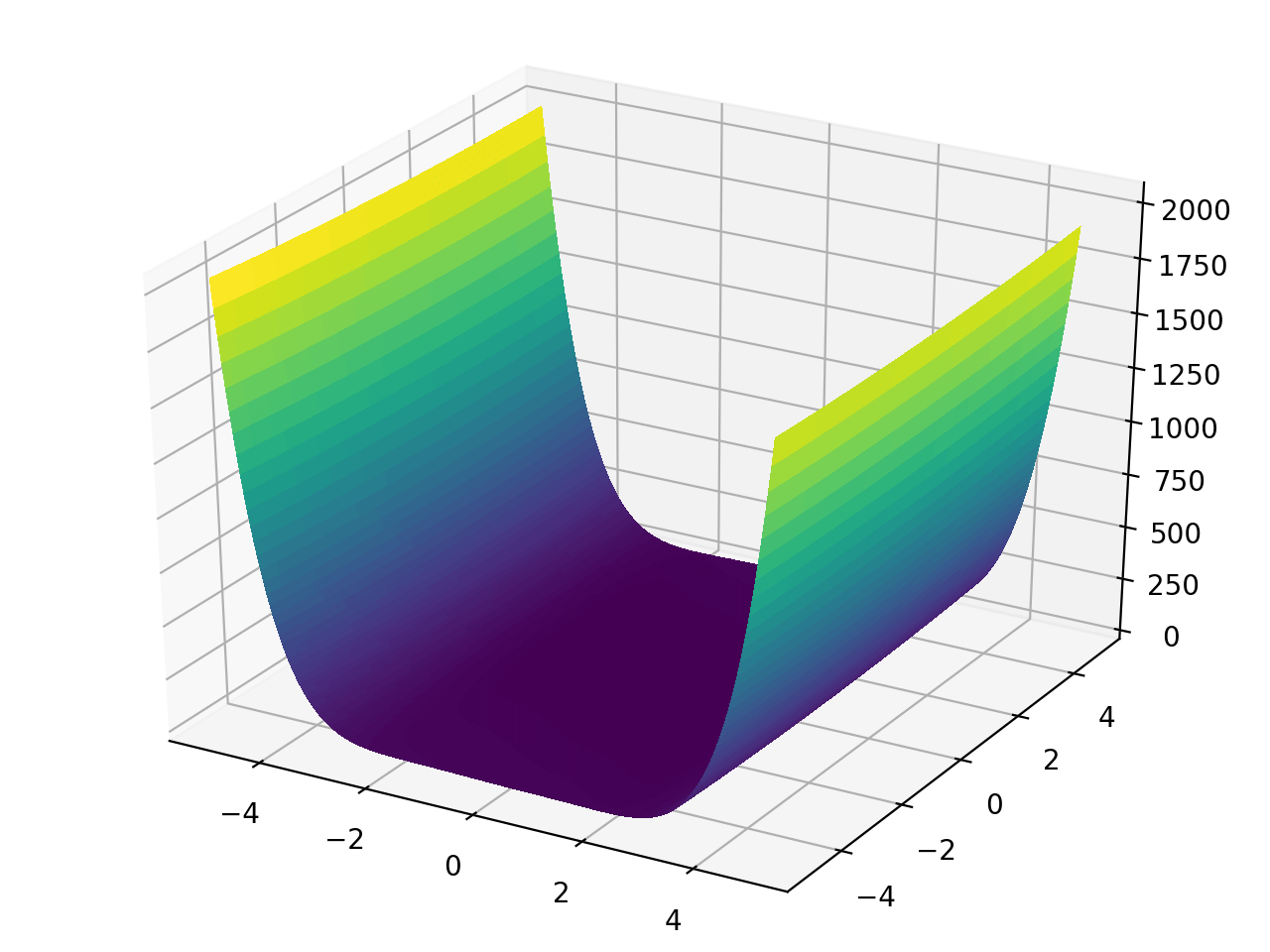### Travelling salesman problem (TSP)

from landscapes.single_objective import tsp


There are several ways to use the TSP function within Landscapes, all of which involve specifying a list of tsp stops, and a distance function.

Example 1: Multi-dimensional list of points using Euclidean distance function

from landscapes.single_objective import tsp
from scipy.spatial import distance

np.random.seed(10)
pts = np.random.rand(5,3)


which will yield a list of three-dimensional points:

array([[0.77132064, 0.02075195, 0.63364823],
[0.74880388, 0.49850701, 0.22479665],
[0.19806286, 0.76053071, 0.16911084],
[0.08833981, 0.68535982, 0.95339335],
[0.00394827, 0.51219226, 0.81262096]])


Then, initialize the tsp function:

tsp_cost = tsp(distance.euclidean, close_loop=True).dist


To calculate the total travel distance, simply call the function with the list of points:

tsp_cost(pts)
>>> 3.2043803044101096


The flag close_loop simply specifies whether the distance between the first and last points should be calculated.

Example 2: Specifying points using Latitude and Longitude

Insead of multi-dimensional points in space, let's specify a list of locations based on longitude and latitude then calculate the distances using the inverse Vincenty's formulae which is available in the spatial package [here].

First let's import our Vincenty based distance function and wrap it for easier use.

from spatial import vincenty_inverse as vi

def vi_tsp(p1, p2):
return vi(p1, p2).mi() # output distance in miles


Next, let's specify some locations. Here are some breweries in Cincinnati. Each row represents a [longitude, latitude].

pts = [
[-84.508661, 39.110187],
[-84.520021, 39.117219],
[-84.514938, 39.113937],
[-84.517401, 39.111322],
[-84.476906, 39.128957]]


Again, initialize the tsp function:

tsp_cost = tsp(vi_tsp, close_loop=True).dist


And finally, calculate the travel distance:

tsp_cost(pts)
>>> 5.993331331465468


Example #3: Geospatial distances on a graph

In Example #2 we used Vincenty's inverse formulae which calculates the distance between two longitude and latitude pairs "as the crow flies". That's great for some situations, but in a city where we're limited by streets and sidewalks, it's a little less useful. Instead, what we want is the actual distance if we were going to walk or bike. This is the network distance and is only slighly more complex, but involves some additinal libraries.

First, import the dependencies:

import osmnx as ox
import networkx as nx
import pandas as pd


Next, load the brewery locations (available here) and prepare the Open Street Map (OSM) network graph.

pts_df = pd.read_csv('brewery_locations.csv')

# determine bounds for osm network
lats = locs_df['lat'].values
lngs = locs_df['lng'].values

bbox = [
max(lats) + 0.1,
min(lats) - 0.1,
max(lngs) + 0.1,
min(lngs) - 0.1]

G = ox.graph_from_bbox(bbox, bbox, bbox, bbox, network_type='drive')


Downloading the osm graph might take a bit depending on internet speed. Next, let's create a new cost function that takes in two brewery names and returns the network distance in meters.

def osm_dist(n0, n1):
p0 = pts_df[pts_df['name']==n0][['lat','lng']].values
p1 = pts_df[pts_df['name']==n1][['lat','lng']].values

p0_node = ox.get_nearest_node(G, p0)
p1_node = ox.get_nearest_node(G, p1)

dist_m = nx.shortest_path_length(G, p0_node, p1_node, weight='length')
return dist_m


Again, specify the tsp cost function:

tsp_cost = tsp(osm_dist, close_loop=True).dist


And to get the network distance:

tsp_cost(locs_df['name'].values)
>>> 75950.73399999998


This translates to roughly 47 miles.

## Project details

This version0.0.120.0.110.0.100.0.80.0.70.0.60.0.40.0.30.0.20.0.1

Uploaded source
Uploaded py3